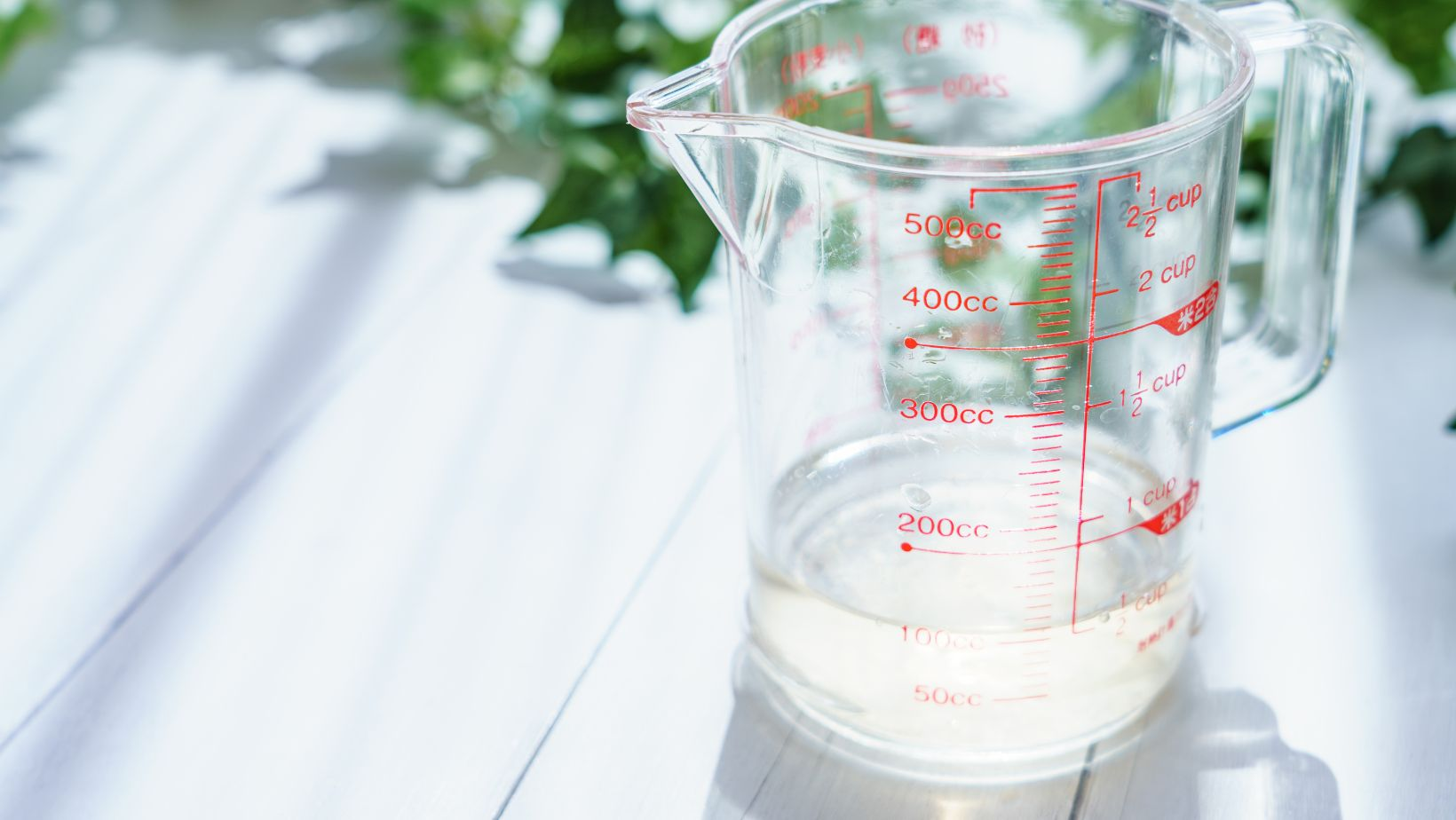# Everything You Need to Know about How Many Ml Are in a Kl## How Many Ml Are in a Kl

Confused about the conversion between millilitres (ml) and kiloliters (kl)? Well, let me break it down for you. When it comes to measuring liquid volume, there are 1,000 millilitres in a litre (L), and 1,000 litres in a kiloliter (kl). Therefore, there are a whopping 1 million millilitres in just one kiloliter!

To put it into perspective, imagine filling up a small glass with water. That would be roughly around 250 ml or a quarter of a litre. Now imagine filling up an Olympic-sized swimming pool. That would contain about 2.5 million litres or 2,500 kl! Quite the difference, isn’t it?

So whether you’re dealing with smaller quantities like millilitres or larger volumes like kiloliters, remember that every kiloliter contains an impressive amount of one million millilitres. It’s always helpful to have this conversion handy when working with different units of liquid volume.

## Conversion Factors for Millilitres to Kiloliters

When converting millilitres (ml) to kiloliters (kl), there are a few factors that need to be taken into consideration. The primary factor is the relationship between these two units of measurement. A kiloliter is equal to 1,000 litres, and a litre is equal to 1,000 millilitres. Therefore, one kiloliter is equivalent to one million millilitres.

To convert millilitres to kiloliters, it’s important to remember that you need to divide the number of millilitres by 1 million. This conversion factor allows you to scale down the volume from a smaller unit (millilitre) to a larger unit (kiloliter). For example:

• If you have 500,000 millilitres, dividing this number by 1 million will give you 0.5 kiloliters.
• Similarly, if you have 2 million millilitres, dividing this number by 1 million will give you 2 kiloliters.

Accurate conversion factors play a crucial role in various fields where precise measurements are required. Whether it’s in scientific research, pharmaceuticals, or manufacturing industries dealing with liquids or volumes on a large scale, having accurate conversions from millilitres to kiloliters ensures consistency and reliable data analysis.

Using incorrect conversion factors can lead to errors in calculations and may result in inaccurate measurements or dosages. It’s essential for professionals working with volumes and liquids regularly to understand and apply the correct conversion factors when converting between different units.

## Converting Millilitres to Kiloliters: A Step-by-Step Guide

Converting millilitre Practical Examples of Converting Millilitres to Kiloliters

When it comes to converting millilitres (ml) to kiloliters (kl), it’s important to understand the relationship between these two units of measurement. A kiloliter is equal to 1,000 litres, while a millilitre is one-thousandth of a litre. So, how many millilitres are in a kiloliter? The answer is 1,000,000 ml.

To help you grasp this concept further, let’s dive into some practical examples that demonstrate the conversion from millilitres to kiloliters:

1. Example 1: Cooking Measurement Imagine you’re following a recipe that requires you to use 2 litres of water. If you need to convert this amount into kiloliters, simply divide it by 1,000 since there are 1,000 litres in a kiloliter. Therefore, converting 2 litres gives us:

2 litres ÷ 1,000 = 0.002 kl

So in this case, we would have 0.002 kiloliters or 2,000 millilitres.

1. Example 2: Beverage Production Suppose a beverage company produces bottles containing 500 millilitres each and wants to determine the volume of liquid they produce in terms of kiloliters. To find the total volume in kl, divide the total number of bottles by their individual volume and then divide by 1,000:

(10,000 bottles × 500 ml) ÷ (1 litre/1000 ml) ÷ (1000 ml/kl) = 5 kl

In this scenario, the beverage company would be producing a total volume of approximately five kiloliters.

1. Example 3: Swimming Pool Capacity If you have an Olympic-sized swimming pool with a capacity of 2,500,000 litres, you can convert this volume into kiloliters by dividing it by 1,000:

2,500,000 litres ÷ 1,000 = 2,500 kl

This means the Olympic-sized swimming pool has a capacity of 2,500 kiloliters or 2.5 million millilitres.

Remember that converting from millilitres to kiloliters involves dividing the number of millilitres by one million (1,000,000) since there are one million millilitres in a kiloliter. By using these practical examples as references, you can easily understand and apply the conversion from millilitres to kiloliters in various real-life scenarios.

Amanda is the proud owner and head cook of her very own restaurant. She loves nothing more than experimenting with new recipes in the kitchen, and her food is always a big hit with customers. Amanda takes great pride in her work, and she always puts her heart into everything she does. She's a hard-working woman who has made it on her own, and she's an inspiration to all who know her.